# 编程能力 —— 寻路问题wendraw

﻿

﻿

# 1. 画迷宫

<style></style><div id="container"></div><script>  let container = document.getElementById('container');  for (let y = 0; y < 100; y++) {    for (let x = 0; x < 100; x++) {      let cell = document.createElement('div');      cell.classList.add("cell");      container.appendChild(cell);    }  }</script>

﻿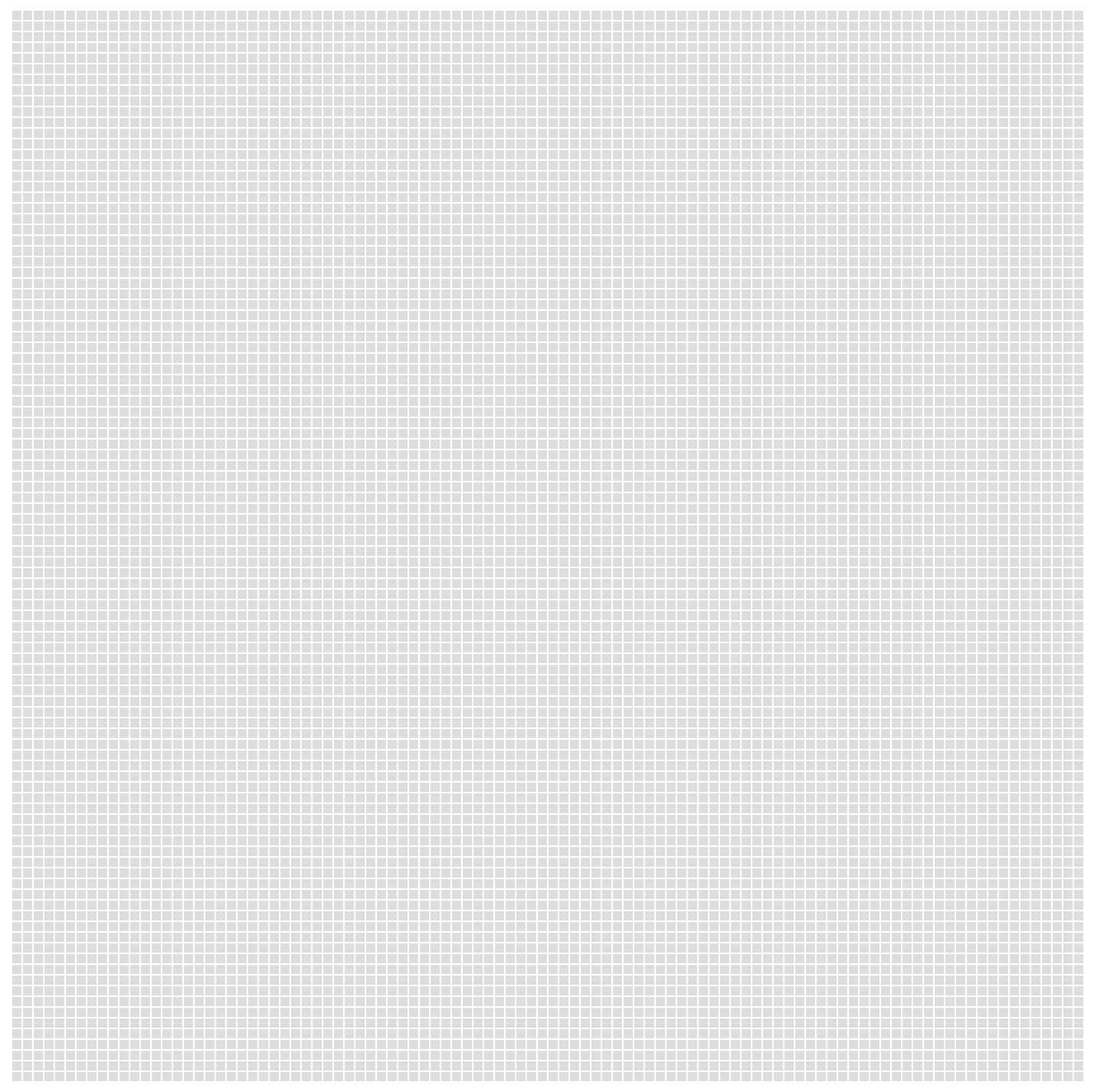image.png

﻿

## 鼠标绘制

﻿

<script>  const map = new Array(10000).fill(0);  const container = document.getElementById('container');  function show(map) {    let move = false;    let clear = false;    container.addEventListener('mousedown', e => {      move = true;      clear = e.button === 2; // 鼠标右键    })    container.addEventListener("mouseup", e => {      move = false;    })    // 取消浏览器右键弹出菜单    container.addEventListener("contextmenu", e => e.preventDefault());    for (let y = 0; y < 100; y++) {      for (let x = 0; x < 100; x++) {        let cell = document.createElement('div');        cell.classList.add("cell");        cell.addEventListener("mousemove", () => {          if (move) {            if (clear) {              cell.style.backgroundColor = "";              map[y * 100 + x] = 0;            } else {              cell.style.backgroundColor = "black";              map[y * 100 + x] = 1;            }          }        });        container.appendChild(cell);        if (map[y * 100 + x] === 1) {          cell.style.backgroundColor = "black";        }      }    }  }  show(map);</script>

﻿

﻿

﻿

﻿

﻿

## 持久化

﻿

﻿

<style></style><div id="container"></div><button onclick="localStorage.map = JSON.stringify(map);">save</button><button onclick="delete localStorage.map;location.reload();">clear</button><script>  let map = localStorage.map ? JSON.parse(localStorage.map) : new Array(10000).fill(0);  // ......</script>

const start = [0, 0];const end = [99, 99];function show() {  // ......  for (let y = 0; y < 100; y++) {    // ......    for (let x = 0; x < 100; x++) {      if (y === 0 && x === 0) {        if (x === start && y === start) {          cell.style.backgroundColor = "yellow";        }        if (x === end && y === end) {          cell.style.backgroundColor = "red";        }      }    }  }

﻿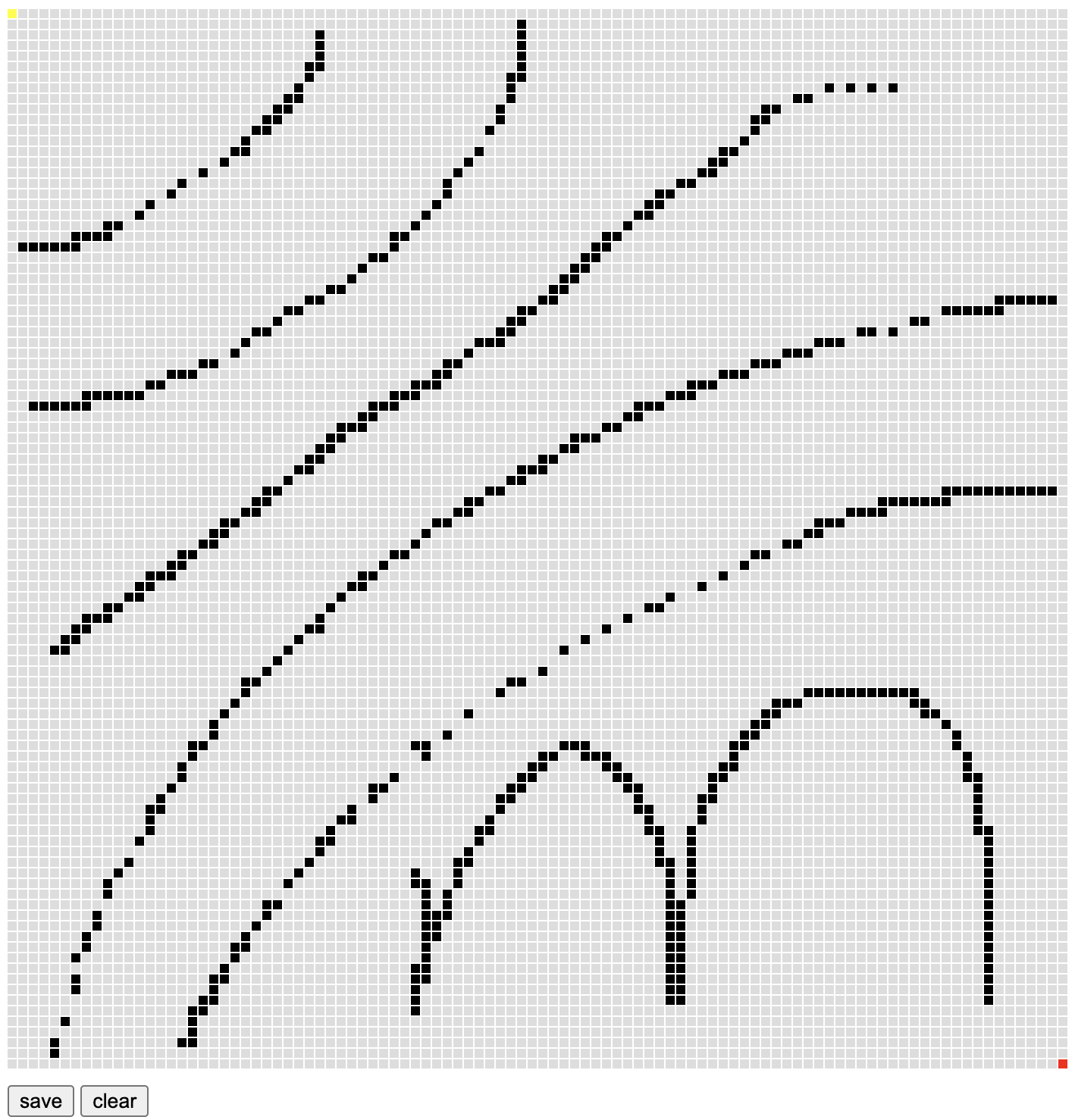image.png

﻿

# 2. BFS

﻿

﻿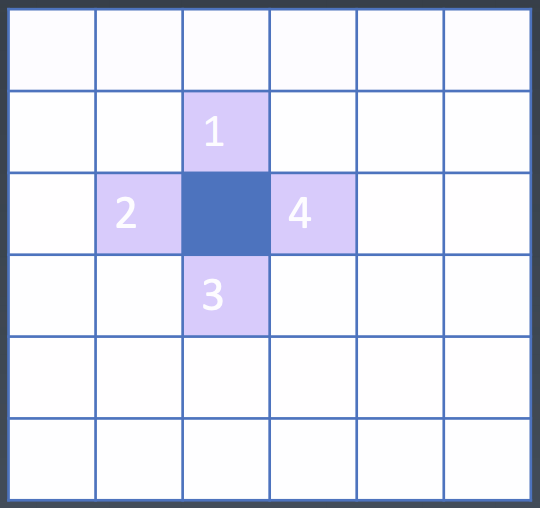image.png

﻿

function findPath(map, start, end) {  let _map = map.slice();  let queue = [start];  while (queue.length) {    let [x, y] = queue.shift();    console.log(x, y);    if (x === end && y === end) {      return true;    }    await insert([x - 1, y]); // 左    await insert([x + 1, y]); // 右    await insert([x, y - 1]); // 上    await insert([x, y + 1]); // 下  }  return false;  function insert([x, y]) {    if (_map[y * 100 + x] !== 0) return;    if (x < 0 || y < 0 || x >= 100 || y >= 100) return;    _map[y * 100 + x] = 2;    queue.push([x, y]);  }}

﻿

BFS 的代码其实非常简单，需要借助队列的特性先入先出，每次出队一个坐标，先判断当前的坐标是不是终点，如果是，就直接结束了。如果不是，就依次将上、下、左、右的坐标入队。当然入队前先要判断是不是障碍物和边界条件，然后再将当前的点标记为 2 表示已经访问过了。这样就会像涟漪一样一圈一圈的往外扩散式的搜索。

﻿

﻿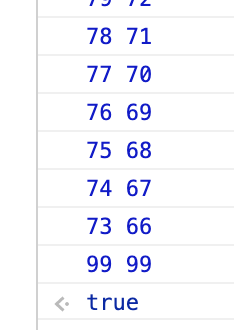image.png

﻿

# 3. 可视化

﻿

﻿

async function findPath(map, start, end) {  let _map = map.slice();  let queue = [start];  while (queue.length) {    let [x, y] = queue.shift();    if (x === end && y === end) {      return true;    }    await insert([x - 1, y]); // 左    await insert([x + 1, y]); // 右    await insert([x, y - 1]); // 上    await insert([x, y + 1]); // 下  }  return false;  async function insert([x, y]) {    if (_map[y * 100 + x] !== 0) return;    if (x < 0 || y < 0 || x >= 100 || y >= 100) return;    _map[y * 100 + x] = 2;    container.children[y * 100 + x].style.backgroundColor = "lightgreen";    await sleep(5);    queue.push([x, y]);  }}function sleep(ms) {  return new Promise((resolve, reject) => {    setTimeout(resolve, ms);  })}

﻿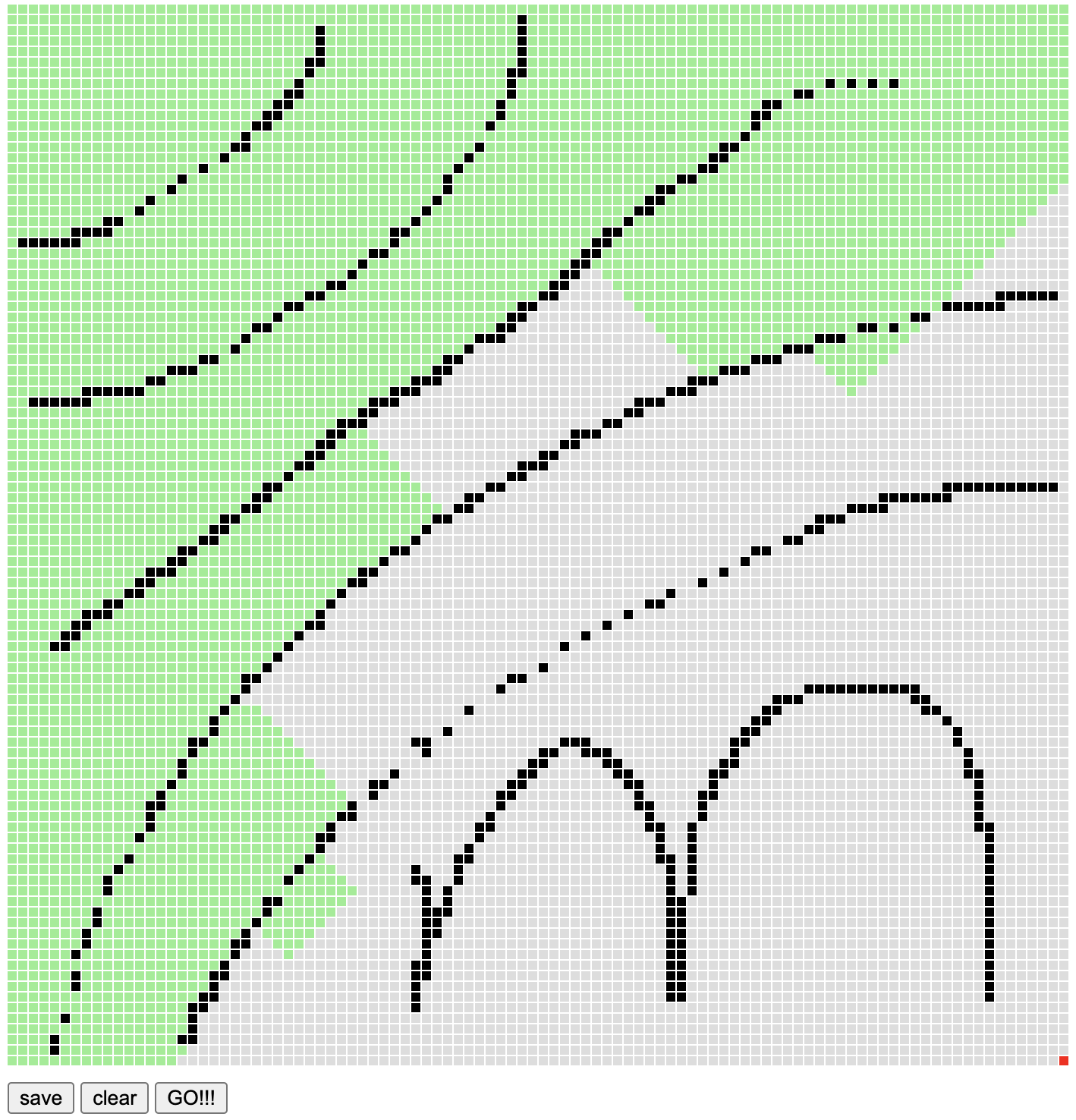image.png

﻿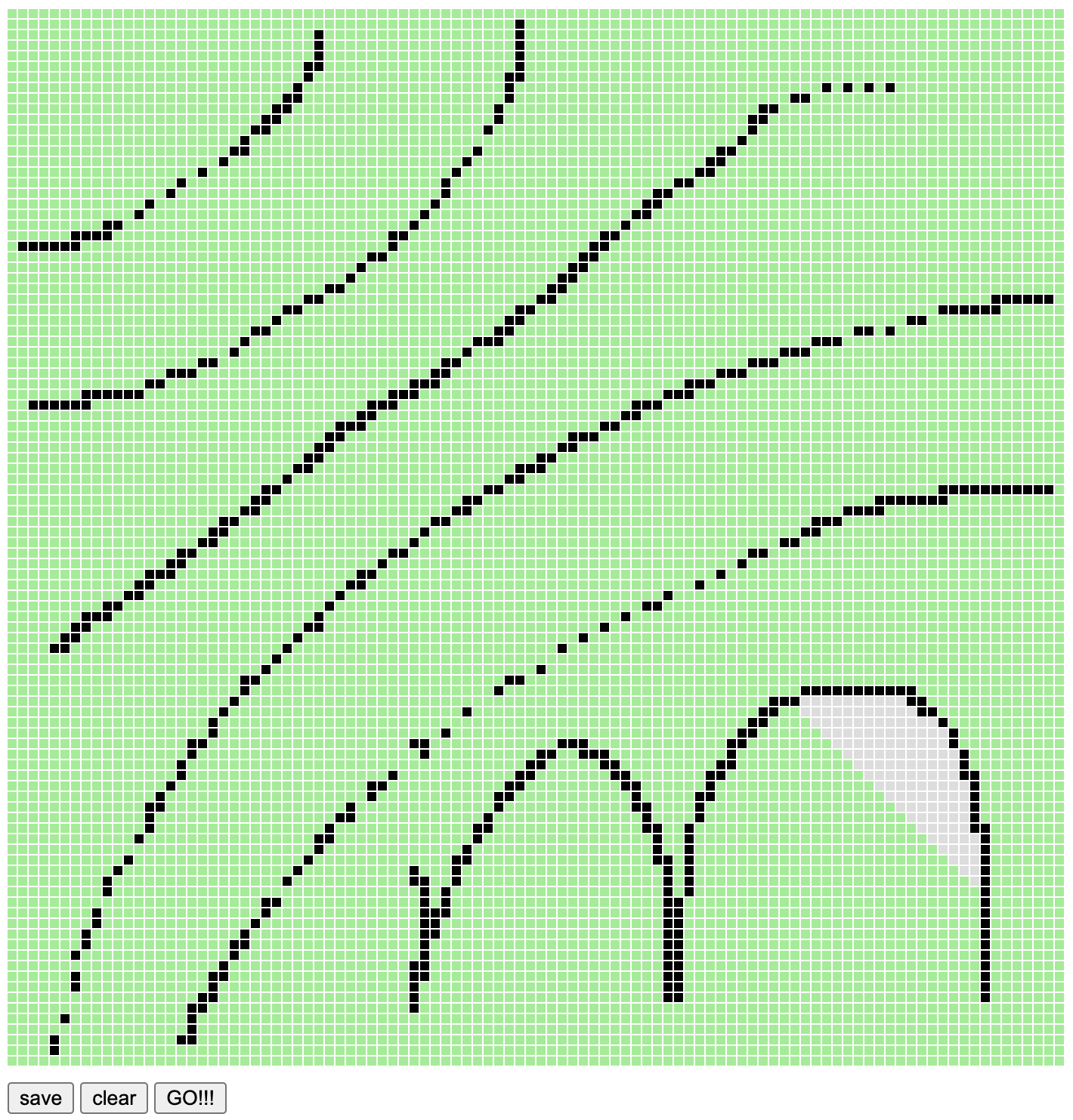image.png

﻿

# 4. 寻路 -- 8 个方向

﻿

async function findPath(map, start, end) {  let _map = map.slice();  let queue = [start];  while (queue.length) {    let [x, y] = queue.shift();    if (x === end && y === end) {      let path = [];      while (x !== start || y !== start) {        path.push([x, y]);        container.children[y * 100 + x].style.backgroundColor = "pink";        [x, y] = _map[y * 100 + x];      }      return path;    }    // ...  }  return null;  async function insert([x, y], pre) {    // ......    _map[y * 100 + x] = pre;    // ......  }}

﻿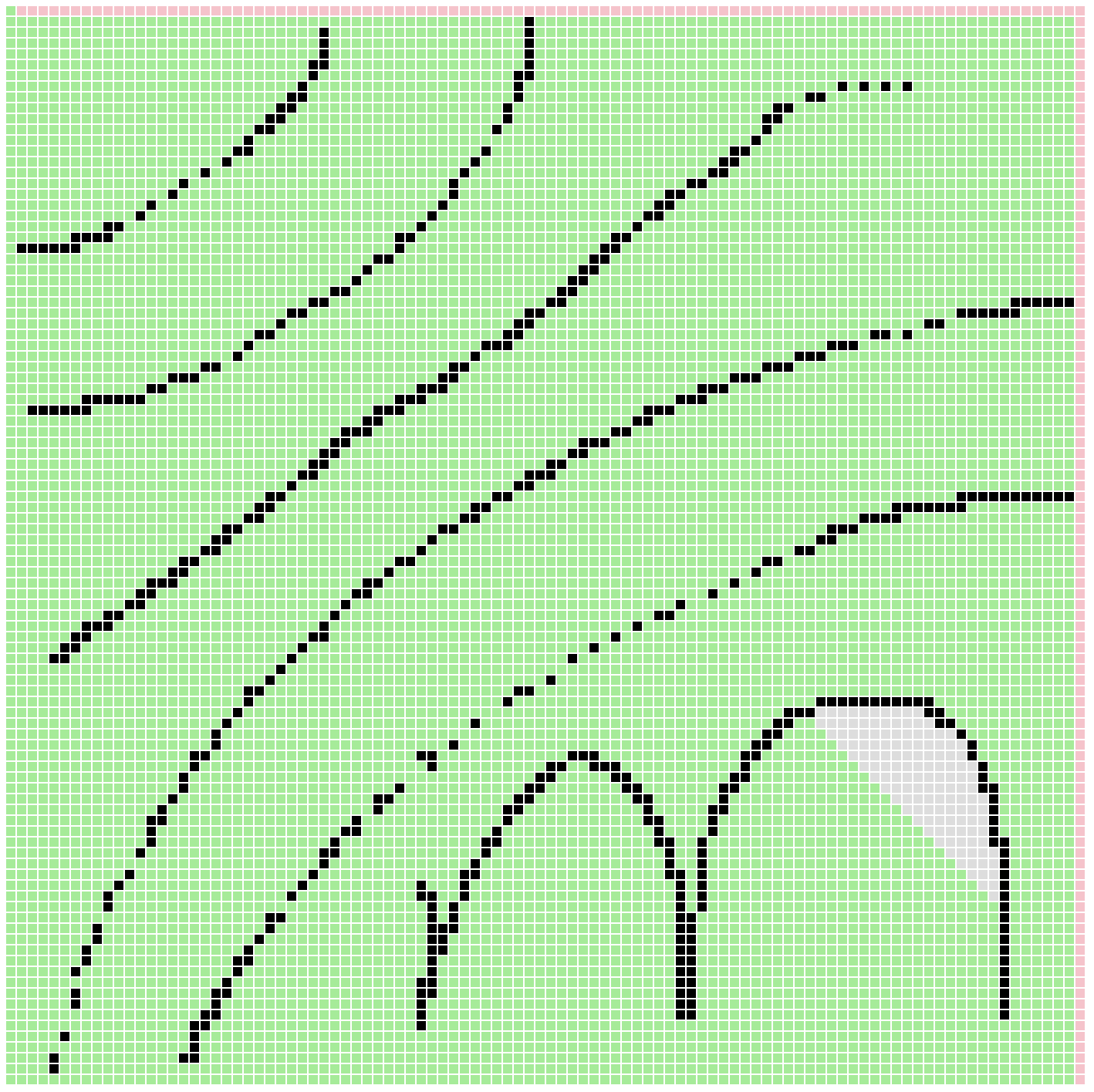image.png

﻿

﻿

async function findPath(map, start, end) {  // ......  while (queue.length) {    // ......    await insert([x - 1, y]); // 左    await insert([x + 1, y]); // 右    await insert([x, y - 1]); // 上    await insert([x, y + 1]); // 下    await insert([x - 1, y - 1]); // 左下    await insert([x - 1, y + 1]); // 左上    await insert([x + 1, y - 1]); // 右下    await insert([x + 1, y + 1]); // 右上  }  // ......}

﻿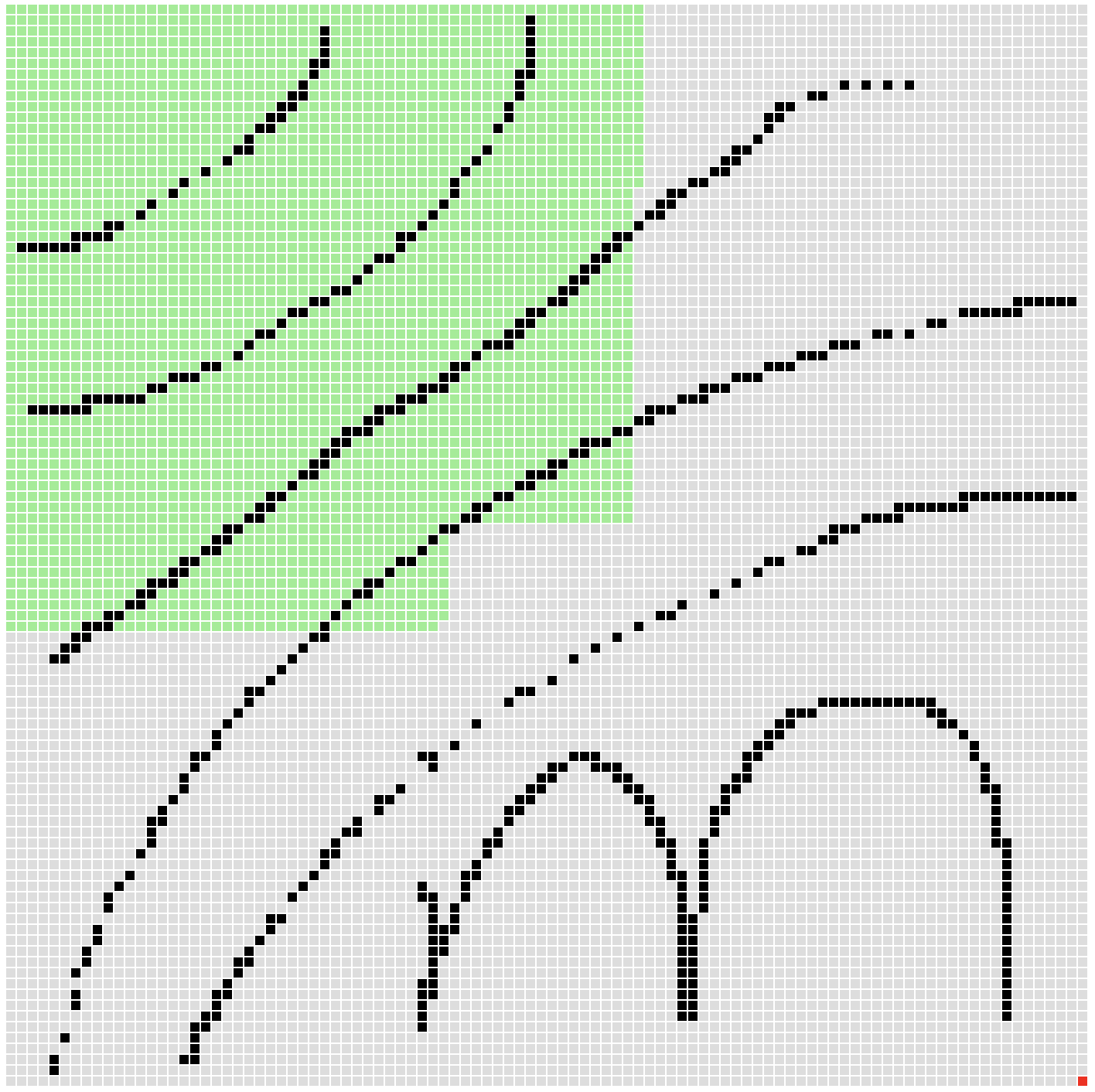image.png

﻿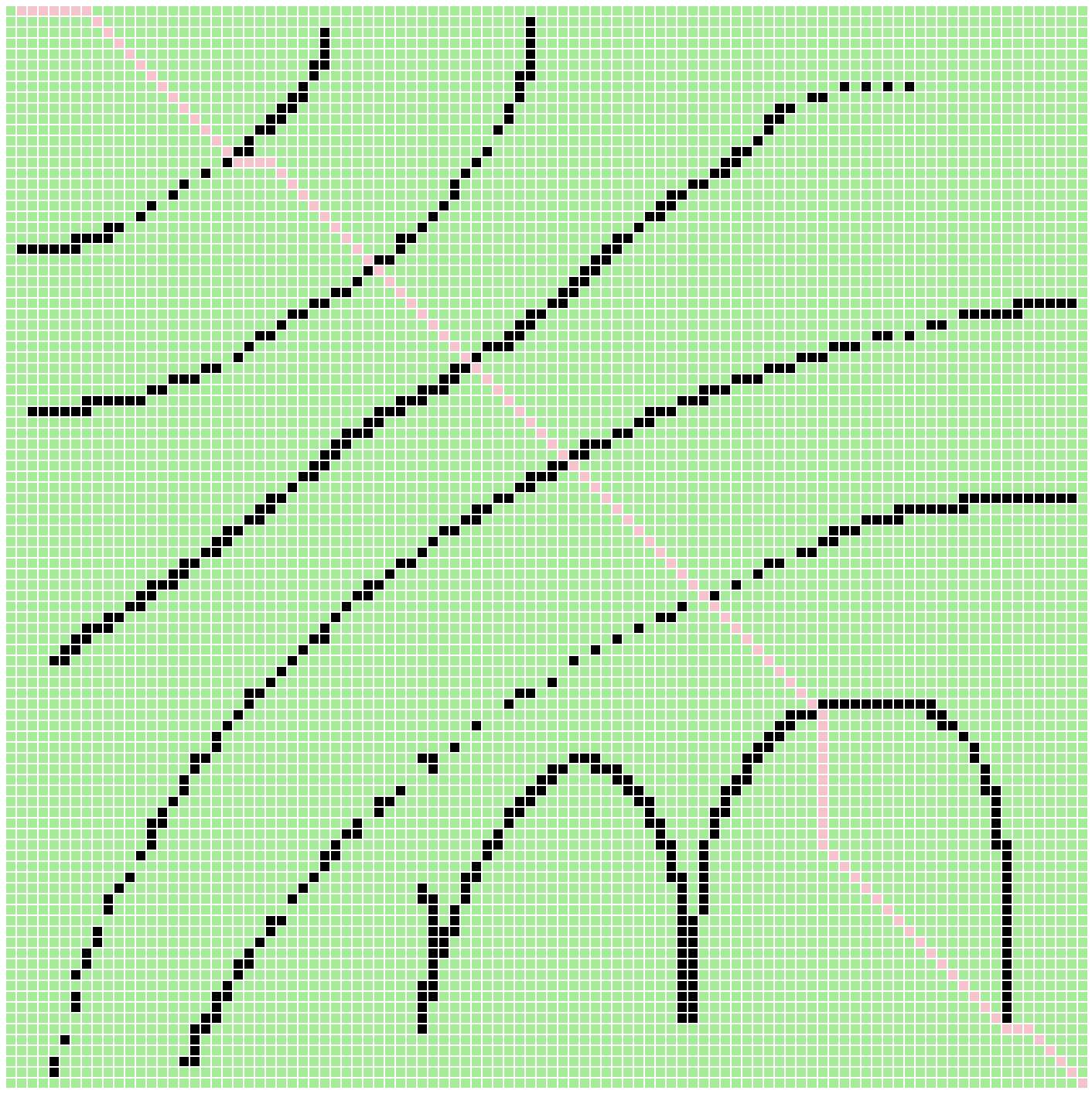image.png

﻿

﻿

(map[y * 100 + (x - 1)] === 0 || map[(y - 1) * 100 + x] === 0) && await insert([x - 1, y - 1], [x, y]); // 左下(map[y * 100 + (x - 1)] === 0 || map[(y + 1) * 100 + x] === 0) && await insert([x - 1, y + 1], [x, y]); // 左上(map[y * 100 + (x + 1)] === 0 || map[(y - 1) * 100 + x] === 0) && await insert([x + 1, y - 1], [x, y]); // 右下(map[y * 100 + (x + 1)] === 0 || map[(y + 1) * 100 + x] === 0) && await insert([x + 1, y + 1], [x, y]); // 右上

﻿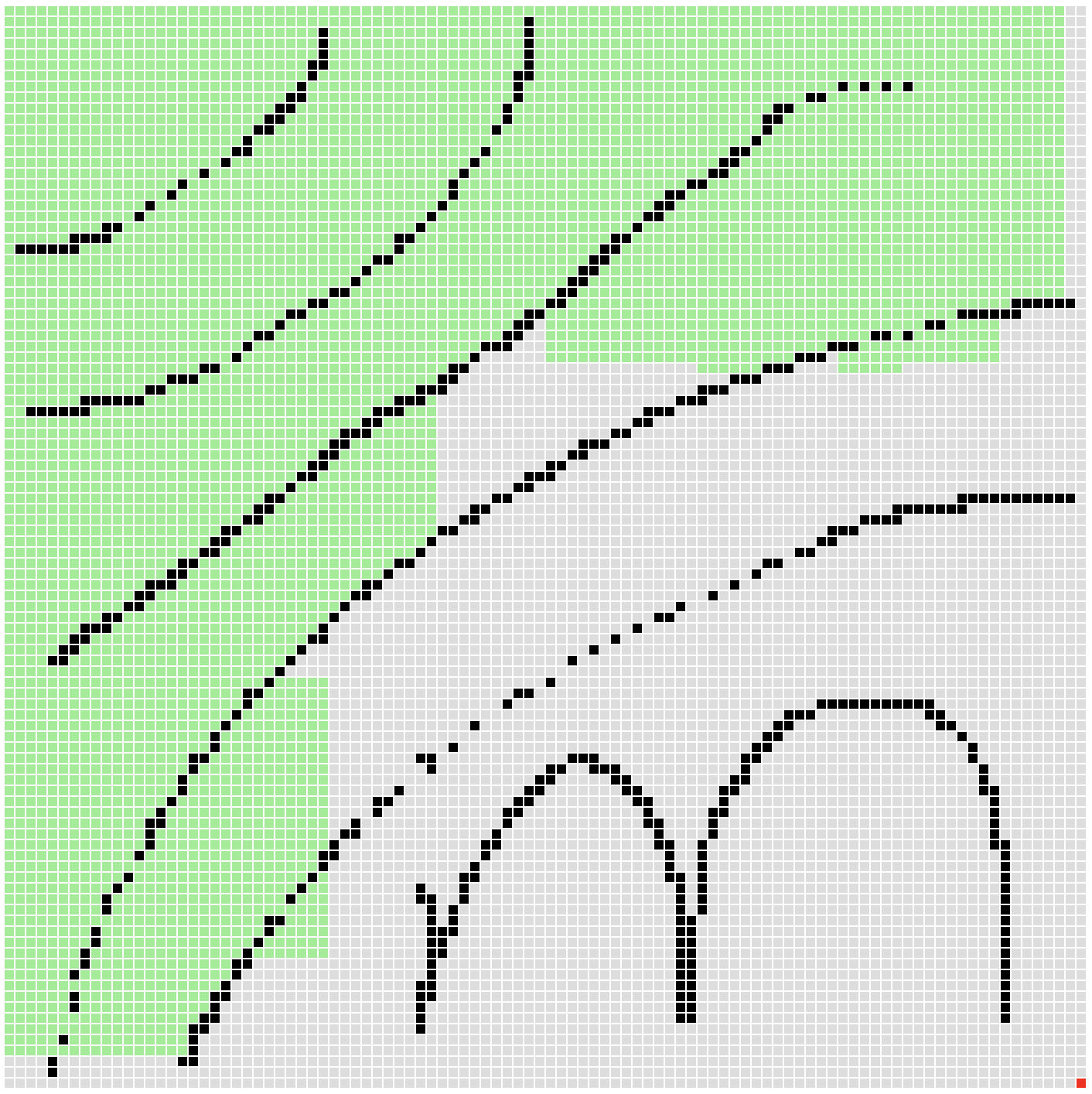image.png

﻿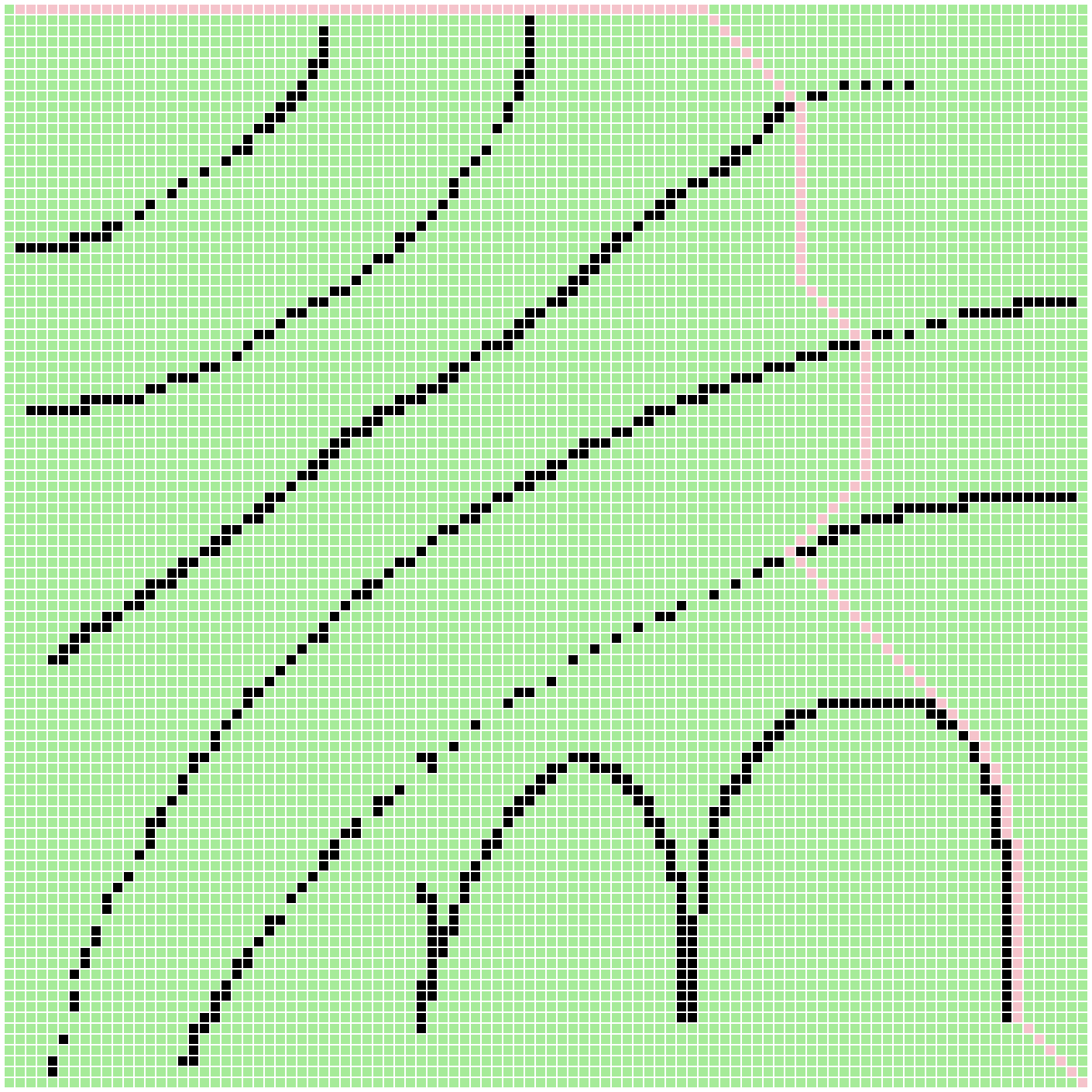image.png

﻿

# 5. 寻路（优化）-- A*

﻿

﻿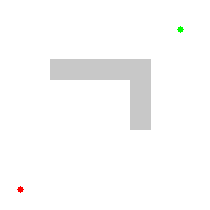﻿

﻿

﻿

class SortedArray {  constructor(data, compare) {    this.data = data;    this.compare = compare;  }  take() {    let min = this.data;    let minIndex = 0;    for (let i = 1; i < this.data.length; i++) {      if (this.compare(min, this.data[i]) > 0) {        min = this.data[i];        minIndex = i;      }    }    this.data[minIndex] = this.data[this.data.length - 1];    this.data.pop();    return min;  }  insert(v) {    this.data.push(v);  }  get length() {    return this.data.length;  }}

﻿

take() 方法也非常简单，每次都在 data 中取最小的那个数据。最小的数被取出之后，就有一个空位，然后我们的数组是无序的，所以只需要将最后一个数填到当前的最小值的空位即可。最后将这个最小值返回。

﻿

insert() 方法只需要将数据插入到数组的末尾。length 是一个 get 方法，这是是为了 BFS 的代码而加上的。

﻿

async function findPath(map, start, end) {  let _map = map.slice();  let collection = new SortedArray([start], (a, b) => distance(a) - distance(b));  while (collection.length) {    let [x, y] = collection.take();    // ......  }  return null;  async function insert([x, y], pre) {    // ......    collection.insert([x, y]);  }  function distance([x, y]) {    return (x - end) ** 2 + (y - end) ** 2;  }}

compare() 算法就使用两点间距离公式来比较，并且我们只涉及大小比较，就可以省去开根号这个耗时的运算。

﻿

﻿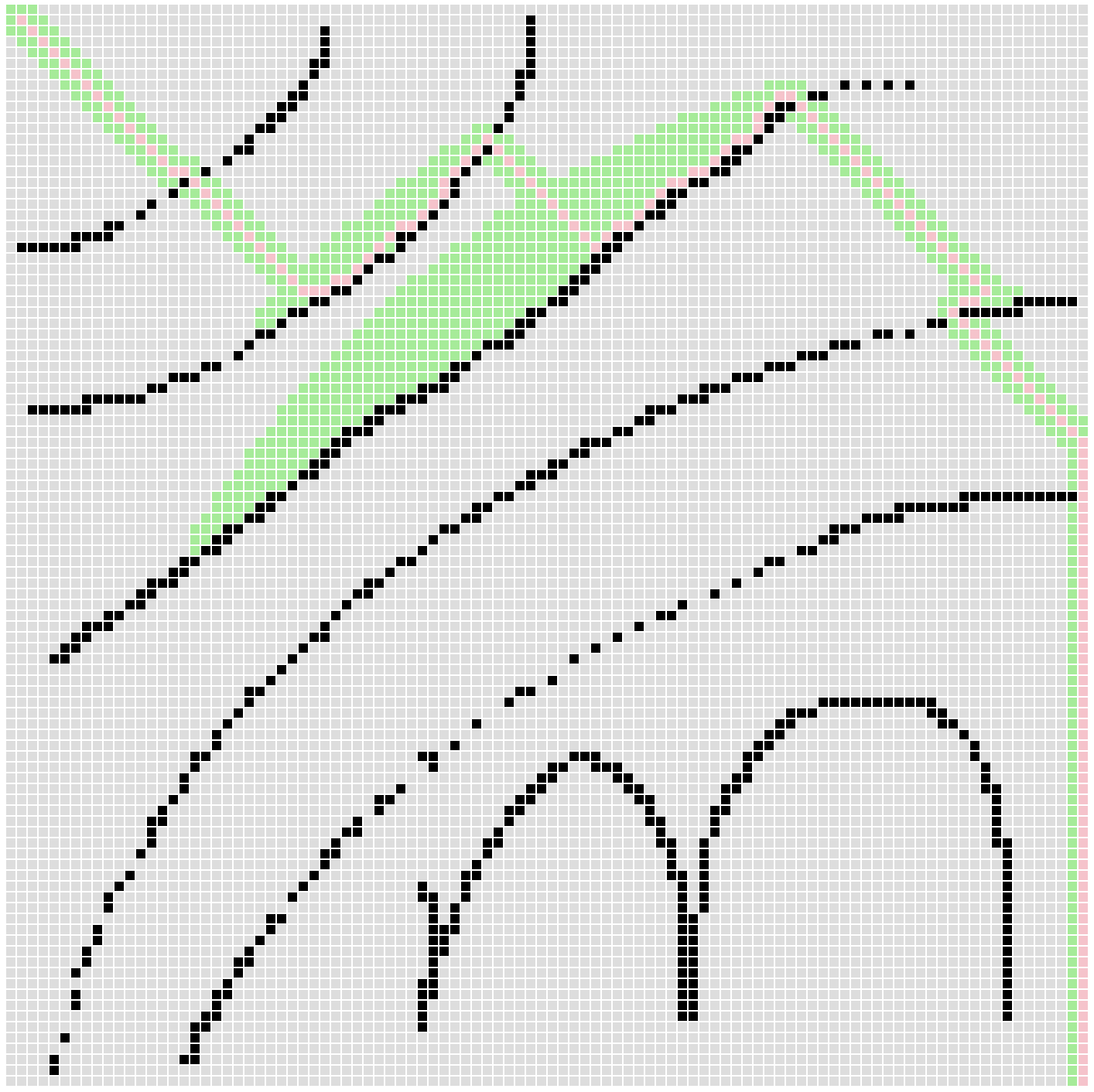image.png

﻿

# 6. 寻路（优化）-- 二叉小顶堆

﻿

﻿

﻿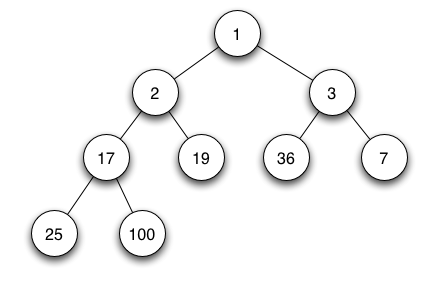image.png

﻿

class BinaryHeap {  constructor(data, compare) {    this.data = data;    this.compare = compare;  }  take() {    if (this.data.length === 0) return;    let min = this.data;    let i = 0;    // fix heap    while (i < this.data.length) {      // 没有左子节点      if (i * 2 + 1 >= this.data.length) break;      // 没有右子节点      if (i * 2 + 2 >= this.data.length) {        this.data[i] = this.data[i * 2 + 1];        i = i * 2 + 1;        break;      }      // 比较左右子节点的大小，更小的补到父节点      if (this.compare(this.data[i * 2 + 1], this.data[i * 2 + 2]) < 0) {        this.data[i] = this.data[i * 2 + 1];        i = i * 2 + 1;      } else {        this.data[i] = this.data[i * 2 + 2];        i = i * 2 + 2;      }    }    if (i < this.data.length - 1) {      this.insertAt(i, this.data.pop());    } else {      this.data.pop();    }    return min;  }  insertAt(i, v) {    this.data[i] = v;    // 对比当前节点与其子节点，如果子节点更小就交换它们    while (this.compare(v, this.data[Math.floor((i - 1) / 2)]) < 0) {      this.data[i] = this.data[Math.floor((i - 1) / 2)];      this.data[Math.floor((i - 1) / 2)] = v;      i = Math.floor((i - 1) / 2);    }  }  insert(v) {    this.insertAt(this.data.length, v);  }  get length() {    return this.data.length;  }}

﻿

﻿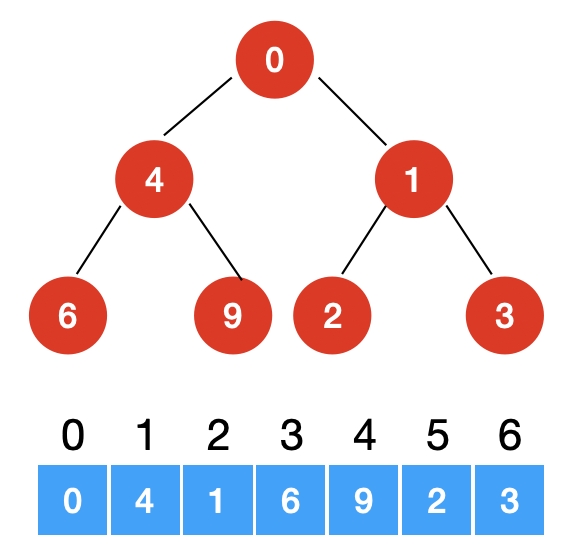image.png

﻿

﻿

insert() 方法就是每次在数组的末尾 insertAt 一位数据。

﻿

insertAt() 方法就是将数值放到指定的位置，但同时还要保证最小堆的特性。就是要与左右子节点比较，如果子节点更小就交换它们的值。一直循环这个过程，直到子节点都比它大，或者没有子节点（下沉到了叶子节点）。

﻿

take() 每次都会从堆顶取出最小值，这时候堆顶就会产生空位，需要从它的左右子节点选出最小的值来作为根节点（这里不需要在左右子树中找最小节点，因为最小堆的定义就是根节点要比左右子树的所有节点都小），然后左右子节点最小的那个又会有空位，因此就在其左右子节点继续找最小值来填充。循环这个过程，直到空位是在叶子节点就跳出循环。这个时候就判断是不是最后一个叶子节点是空位，如果是，就直接删除即可。如果不是，就将最后一位叶子节点拿出来补上这个空位。

﻿

let collection = new SortedArray([start], (a, b) => distance(a) - distance(b));// 换成let collection = new BinaryHeap([start], (a, b) => distance(a) - distance(b));

﻿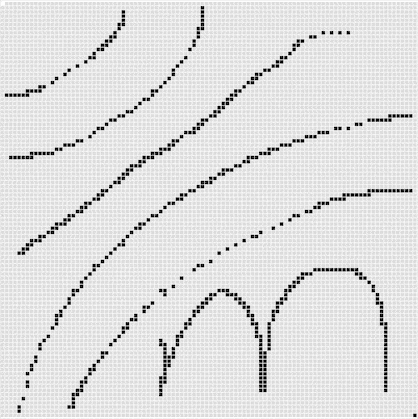﻿

﻿

# 7. 寻路（优化）-- 距离权重

﻿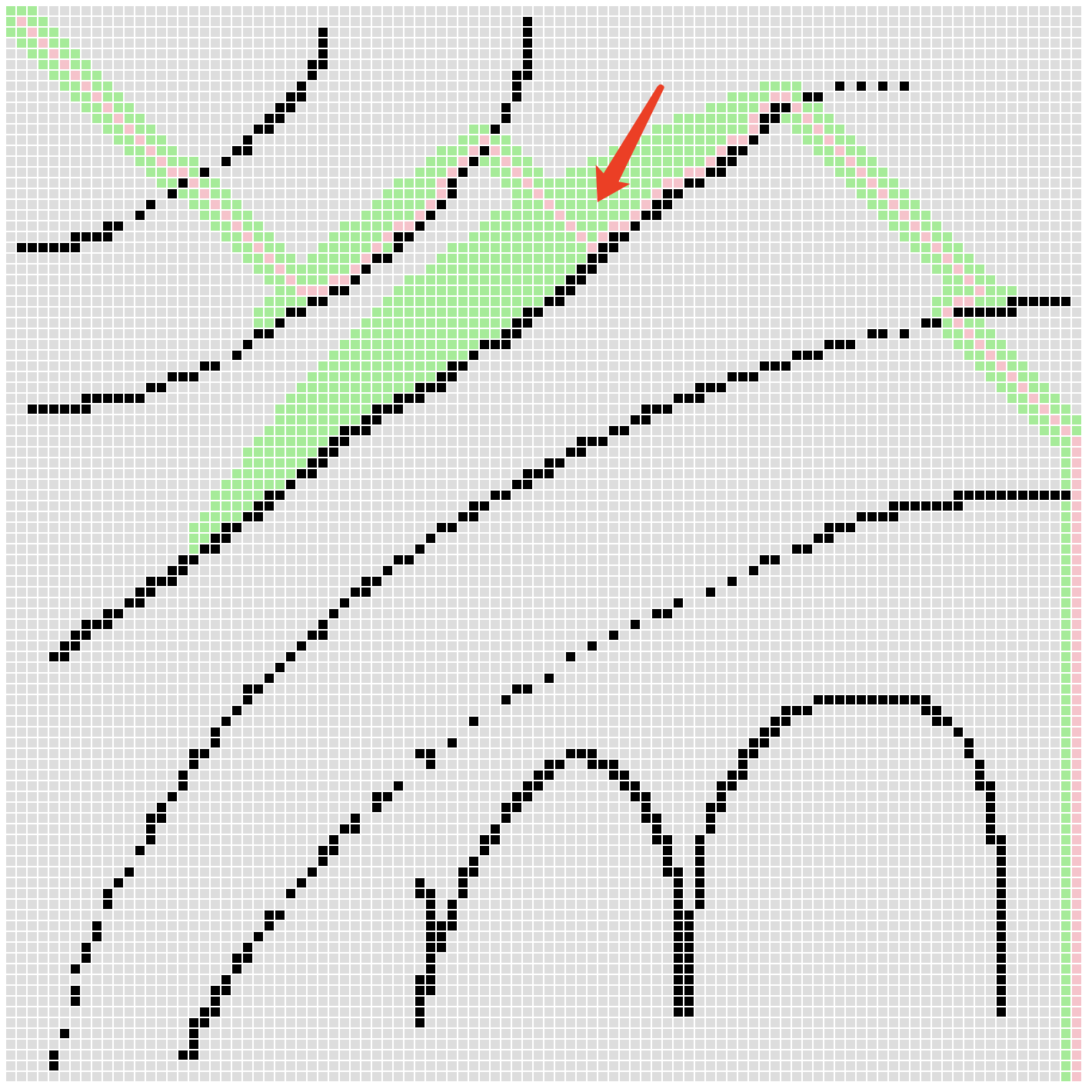image.png

﻿

﻿

async function findPath(map, start, end) {  // ......    // 记录到起点的长度  let table = new Array(10000).fill(Infinity);  table[start * 100 + start] = 0;  while (collection.length) {    let [x, y] = collection.take();    let fromStart = table[y * 100 + x];    // ......    await insert([x - 1, y], [x, y], fromStart + 1); // 左    await insert([x + 1, y], [x, y], fromStart + 1); // 右    await insert([x, y - 1], [x, y], fromStart + 1); // 上    await insert([x, y + 1], [x, y], fromStart + 1); // 下    (map[y * 100 + (x - 1)] === 0 || map[(y - 1) * 100 + x] === 0) && await insert([x - 1, y - 1], [x, y], fromStart + 1.4); // 左下    (map[y * 100 + (x - 1)] === 0 || map[(y + 1) * 100 + x] === 0) && await insert([x - 1, y + 1], [x, y], fromStart + 1.4); // 左上    (map[y * 100 + (x + 1)] === 0 || map[(y - 1) * 100 + x] === 0) && await insert([x + 1, y - 1], [x, y], fromStart + 1.4); // 右下    (map[y * 100 + (x + 1)] === 0 || map[(y + 1) * 100 + x] === 0) && await insert([x + 1, y + 1], [x, y], fromStart + 1.4); // 右上  }  return null;  async function insert([x, y], pre, fromStart) {    if (_map[y * 100 + x] === 1) return;    if (x < 0 || y < 0 || x >= 100 || y >= 100) return;    if (fromStart >= table[y * 100 + x]) return;    _map[y * 100 + x] = pre;    table[y * 100 + x] = fromStart;    container.children[y * 100 + x].style.backgroundColor = "lightgreen";    await sleep(1);    collection.insert([x, y]);  }  // ......}

﻿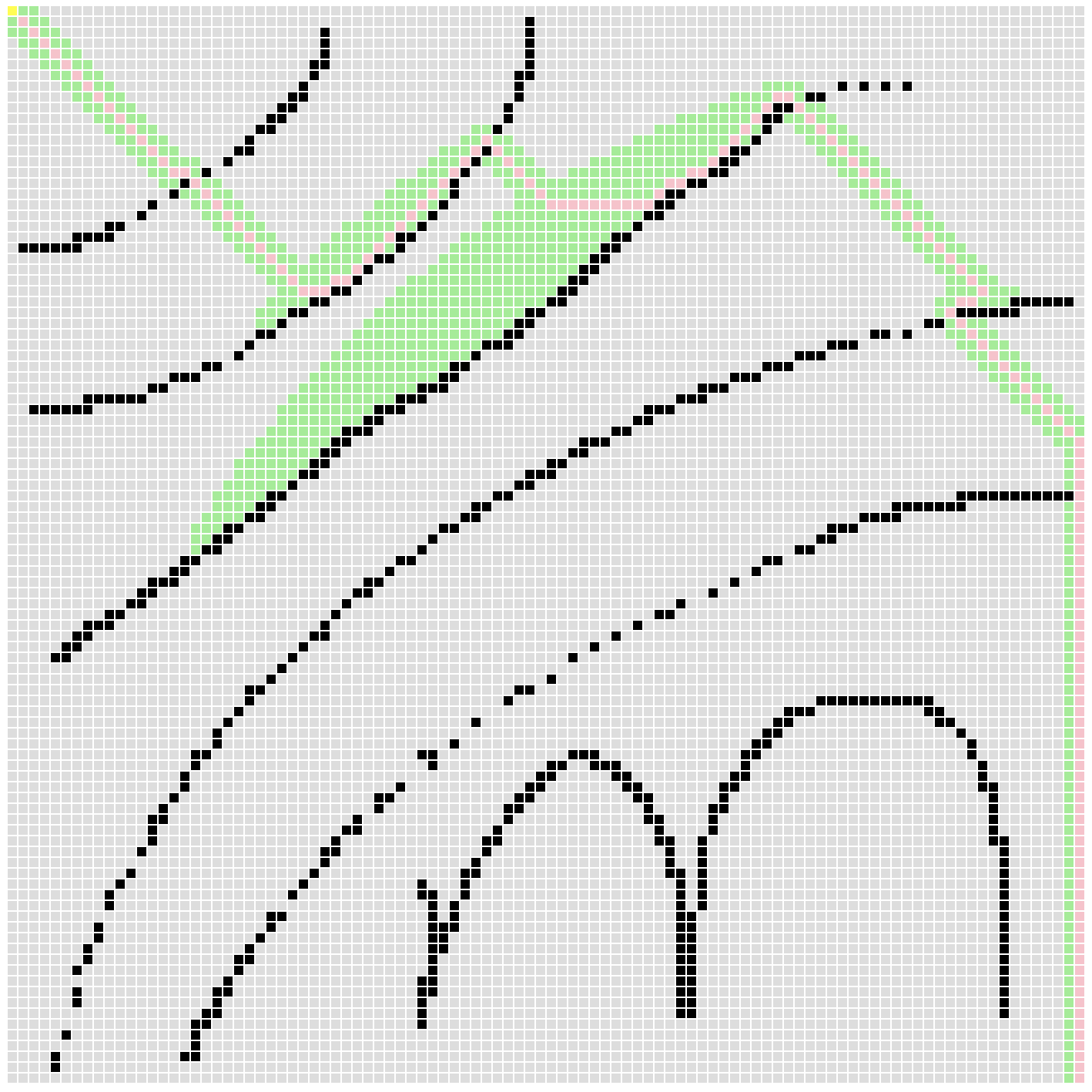image.png

﻿

﻿

﻿

﻿

# 参考

winter 前端进阶训练营

A* search algorithm

28 | 堆和堆排序：为什么说堆排序没有快速排序快？

﻿## 评论